# Train Linear Regression Model

Statistics and Machine Learning Toolbox™ provides several features for training a linear regression model.

• For greater accuracy on low-dimensional through medium-dimensional data sets, use `fitlm`. After fitting the model, you can use the object functions to improve, evaluate, and visualize the fitted model. To regularize a regression, use `lasso` or `ridge`.

• For reduced computation time on high-dimensional data sets, use `fitrlinear`. This function offers useful options for cross-validation, regularization, and hyperparameter optimization.

This example shows the typical workflow for linear regression analysis using `fitlm`. The workflow includes preparing a data set, fitting a linear regression model, evaluating and improving the fitted model, and predicting response values for new predictor data. The example also describes how to fit and evaluate a linear regression model for tall arrays.

### Prepare Data

Load the sample data set `NYCHousing2015`.

`load NYCHousing2015`

The data set includes 10 variable with information on the sales of properties in the New York City in 2015. This example uses some of these variables to analyze the sale prices.

Instead of loading the sample data set `NYCHousing2015`, you can download the data from the NYC Open Data website and import the data as follows.

```folder = 'Annualized_Rolling_Sales_Update'; ds = spreadsheetDatastore(folder,"TextType","string","NumHeaderLines",4); ds.Files = ds.Files(contains(ds.Files,"2015")); ds.SelectedVariableNames = ["BOROUGH","NEIGHBORHOOD","BUILDINGCLASSCATEGORY","RESIDENTIALUNITS", ... "COMMERCIALUNITS","LANDSQUAREFEET","GROSSSQUAREFEET","YEARBUILT","SALEPRICE","SALEDATE"]; NYCHousing2015 = readall(ds); ```

Preprocess the data set to choose the predictor variables of interest. First, change the variable names to lowercase for readability.

`NYCHousing2015.Properties.VariableNames = lower(NYCHousing2015.Properties.VariableNames);`

Next, convert the `saledate` variable, specified as a `datetime` array, into two numeric columns `MM` (month) and `DD` (day), and remove the `saledate` variable. Ignore the year values because all samples are for the year 2015.

```[~,NYCHousing2015.MM,NYCHousing2015.DD] = ymd(NYCHousing2015.saledate); NYCHousing2015.saledate = [];```

The numeric values in the `borough` variable indicate the names of the boroughs. Change the variable to a categorical variable using the names.

```NYCHousing2015.borough = categorical(NYCHousing2015.borough,1:5, ... ["Manhattan","Bronx","Brooklyn","Queens","Staten Island"]);```

The `neighborhood` variable has 254 categories. Remove this variable for simplicity.

`NYCHousing2015.neighborhood = [];`

Convert the `buildingclasscategory` variable to a categorical variable, and explore the variable by using the `wordcloud` function.

```NYCHousing2015.buildingclasscategory = categorical(NYCHousing2015.buildingclasscategory); wordcloud(NYCHousing2015.buildingclasscategory);```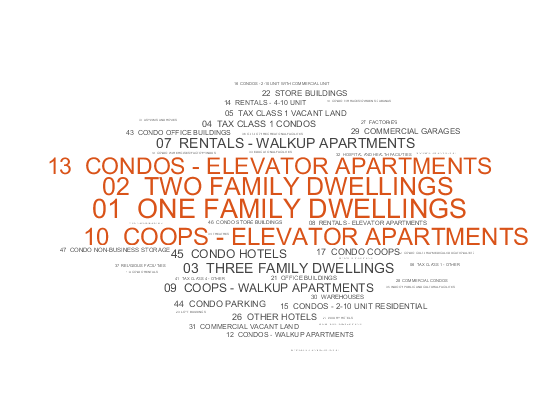Assume that you are interested only in one-, two-, and three-family dwellings. Find the sample indices for these dwellings and delete the other samples. Then, change the data type of the `buildingclasscategory` variable to `double`.

```idx = ismember(string(NYCHousing2015.buildingclasscategory), ... ["01 ONE FAMILY DWELLINGS","02 TWO FAMILY DWELLINGS","03 THREE FAMILY DWELLINGS"]); NYCHousing2015 = NYCHousing2015(idx,:); NYCHousing2015.buildingclasscategory = renamecats(NYCHousing2015.buildingclasscategory, ... ["01 ONE FAMILY DWELLINGS","02 TWO FAMILY DWELLINGS","03 THREE FAMILY DWELLINGS"], ... ["1","2","3"]); NYCHousing2015.buildingclasscategory = double(NYCHousing2015.buildingclasscategory);```

The `buildingclasscategory` variable now indicates the number of families in one dwelling.

Explore the response variable `saleprice` using the `summary` function.

```s = summary(NYCHousing2015); s.saleprice```
```ans = struct with fields: Size: [37881 1] Type: 'double' Description: '' Units: '' Continuity: [] Min: 0 Median: 352000 Max: 37000000 NumMissing: 0 ```

Assume that a `saleprice` less than or equal to \$1000 indicates ownership transfer without a cash consideration. Remove the samples that have this `saleprice`.

```idx0 = NYCHousing2015.saleprice <= 1000; NYCHousing2015(idx0,:) = [];```

Create a histogram of the `saleprice` variable.

`histogram(NYCHousing2015.saleprice)`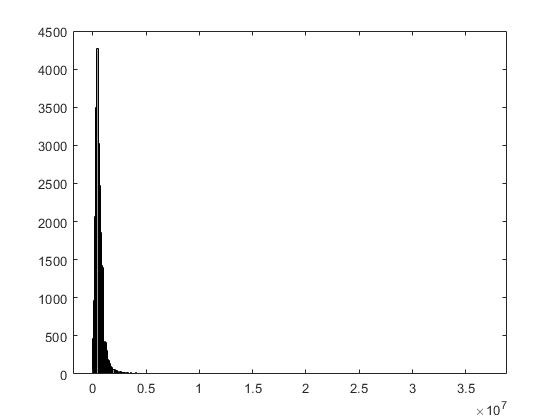The maximum value of `saleprice` is $3.7×1{0}^{7}$, but most values are smaller than $0.5×1{0}^{7}$. You can identify the outliers of `saleprice` by using the `isoutlier` function.

`idx = isoutlier(NYCHousing2015.saleprice);`

Remove the identified outliers and create the histogram again.

```NYCHousing2015(idx,:) = []; histogram(NYCHousing2015.saleprice)```Partition the data set into a training set and test set by using `cvpartition`.

```rng('default') % For reproducibility c = cvpartition(height(NYCHousing2015),"holdout",0.3); trainData = NYCHousing2015(training(c),:); testData = NYCHousing2015(test(c),:);```

### Train Model

Fit a linear regression model by using the `fitlm` function.

```mdl = fitlm(trainData,"PredictorVars",["borough","grosssquarefeet", ... "landsquarefeet","buildingclasscategory","yearbuilt","MM","DD"], ... "ResponseVar","saleprice")```
```mdl = Linear regression model: saleprice ~ [Linear formula with 8 terms in 7 predictors] Estimated Coefficients: Estimate SE tStat pValue ___________ __________ ________ ___________ (Intercept) 2.0345e+05 1.0308e+05 1.9736 0.048441 borough_Bronx -3.0165e+05 56676 -5.3224 1.0378e-07 borough_Brooklyn -41160 56490 -0.72862 0.46624 borough_Queens -91136 56537 -1.612 0.10699 borough_Staten Island -2.2199e+05 56726 -3.9134 9.1385e-05 buildingclasscategory 3165.7 3510.3 0.90185 0.36715 landsquarefeet 13.149 0.84534 15.555 3.714e-54 grosssquarefeet 112.34 2.9494 38.09 8.0393e-304 yearbuilt 100.07 45.464 2.201 0.02775 MM 3850.5 543.79 7.0808 1.4936e-12 DD -367.19 207.56 -1.7691 0.076896 Number of observations: 15848, Error degrees of freedom: 15837 Root Mean Squared Error: 2.32e+05 R-squared: 0.235, Adjusted R-Squared: 0.235 F-statistic vs. constant model: 487, p-value = 0 ```

`mdl` is a `LinearModel` object. The model display includes the model formula, estimated coefficients, and summary statistics.

`borough` is a categorical variable that has five categories: `Manhattan`, `Bronx`, `Brooklyn`, `Queens`, and `Staten Island`. The fitted model `mdl` has four indicator variables. The `fitlm` function uses the first category `Manhattan` as a reference level, so the model does not include the indicator variable for the reference level. `fitlm` fixes the coefficient of the indicator variable for the reference level as zero. The coefficient values of the four indicator variables are relative to `Manhattan`. For more details on how the function treats a categorical predictor, see Algorithms of `fitlm`.

To learn how to interpret the values in the model display, see Interpret Linear Regression Results.

You can use the properties of a `LinearModel` object to investigate a fitted linear regression model. The object properties include information about coefficient estimates, summary statistics, fitting method, and input data. For example, you can find the R-squared and adjusted R-squared values in the `Rsquared` property. You can access the property values through the Workspace browser or using dot notation.

`mdl.Rsquared`
```ans = struct with fields: Ordinary: 0.2352 Adjusted: 0.2348 ```

The model display also shows these values. The R-squared value indicates that the model explains approximately 24% of the variability in the response variable. See Properties of a `LinearModel` object for details about other properties.

### Evaluate Model

The model display shows the p-value of each coefficient. The p-values indicate which variables are significant to the model. For the categorical predictor `borough`, the model uses four indicator variables and displays four p-values. To examine the categorical variable as a group of indicator variables, use the object function `anova`. This function returns analysis of variance (ANOVA) statistics of the model.

`anova(mdl)`
```ans=8×5 table SumSq DF MeanSq F pValue __________ _____ __________ _______ ___________ borough 1.123e+14 4 2.8076e+13 520.96 0 buildingclasscategory 4.3833e+10 1 4.3833e+10 0.81334 0.36715 landsquarefeet 1.3039e+13 1 1.3039e+13 241.95 3.714e-54 grosssquarefeet 7.8189e+13 1 7.8189e+13 1450.8 8.0393e-304 yearbuilt 2.6108e+11 1 2.6108e+11 4.8444 0.02775 MM 2.7021e+12 1 2.7021e+12 50.138 1.4936e-12 DD 1.6867e+11 1 1.6867e+11 3.1297 0.076896 Error 8.535e+14 15837 5.3893e+10 ```

The p-values for the indicator variables `borough_Brooklyn` and `borough_Queens` are large, but the p-value of the `borough` variable as a group of four indicator variables is almost zero, which indicates that the `borough` variable is statistically significant.

The p-values of `buildingclasscategory` and `DD` are larger than 0.05, which indicates that these variables are not significant at the 5% significance level. Therefore, you can consider removing these variables.

You can also use `coeffCI`, `coeefTest`, and `dwTest` to further evaluate the fitted model.

• `coefCI` returns confidence intervals of the coefficient estimates.

• `coefTest` performs a linear hypothesis test on the model coefficients.

• `dwtest` performs the Durbin-Watson test. (This test is used for time series data, so `dwtest` is not appropriate for the housing data in this example.)

### Visualize Model and Summary Statistics

A `LinearModel` object provides multiple plotting functions.

In addition, `plot` creates an added variable plot for the whole model, except the intercept term, if `mdl` includes multiple predictor variables.

`plot(mdl)`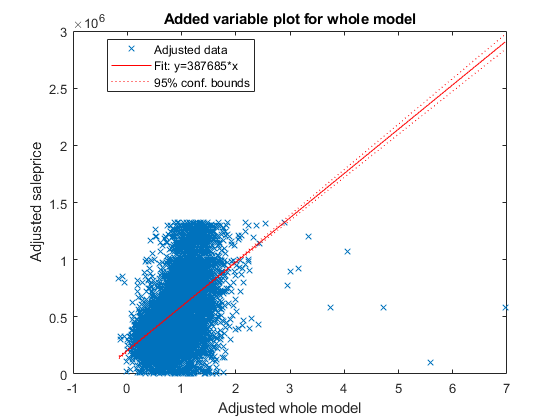This plot is equivalent to `plotAdded(mdl)`. The fitted line represents how the model, as a group of variables, can explain the response variable. The slope of the fitted line is not close to zero, and the confidence bound does not include a horizontal line, indicating that the model fits better than a degenerate model consisting of only a constant term. The test statistic value shown in the model display (`F-statistic vs. constant model`) also indicates that the model fits better than the degenerate model.

Create an added variable plot for the insignificant variables `buildingclasscategory` and `DD`. The p-values of these variables are larger than 0.05. First, find the indices of these coefficients in `mdl.CoefficientNames`.

`mdl.CoefficientNames`
```ans = 1x11 cell Columns 1 through 3 {'(Intercept)'} {'borough_Bronx'} {'borough_Brooklyn'} Columns 4 through 6 {'borough_Queens'} {'borough_Staten...'} {'buildingclassc...'} Columns 7 through 10 {'landsquarefeet'} {'grosssquarefeet'} {'yearbuilt'} {'MM'} Column 11 {'DD'} ```

`buildingclasscategory` and `DD` are the 6th and 11th coefficients, respectively. Create an added plot for these two variables.

`plotAdded(mdl,[6,11])`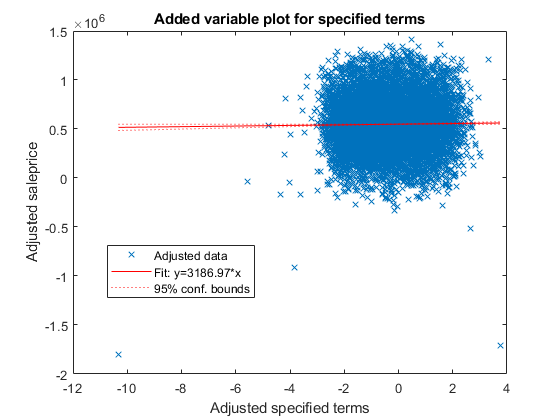The slope of the fitted line is close to zero, indicating that the information from the two variables does not explain the part of the response values not explained by the other predictors. For more details about an added variable plot, see Added Variable Plot.

Create a histogram of the model residuals. `plotResiduals` plots a histogram of the raw residuals using probability density function scaling.

`plotResiduals(mdl)`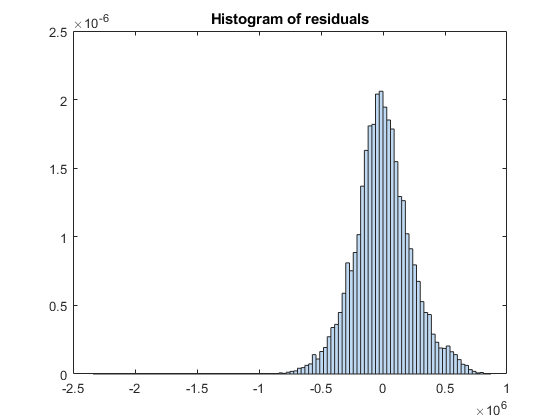The histogram shows that a few residuals are smaller than $-1×1{0}^{6}$. Identify these outliers.

`find(mdl.Residuals.Raw < -1*10^6)`
```ans = 4×1 1327 4136 4997 13894 ```

Alternatively, you can find the outliers by using `isoutlier`. Specify the `'grubbs'` option to apply Grubb's test. This option is suitable for a normally distributed data set.

`find(isoutlier(mdl.Residuals.Raw,'grubbs'))`
```ans = 3×1 1327 4136 4997 ```

The `isoutlier` function does not identify residual `13894` as an outlier. This residual is close to –1$×$10${}^{6}$. Display the residual value.

`mdl.Residuals.Raw(13894)`
```ans = -1.0720e+06 ```

You can exclude outliers when fitting a linear regression model by using the 'Exclude' name-value pair argument. In this case, the example adjusts the fitted model and checks whether the improved model can also explain the outliers.

Remove the `DD` and `buildingclasscategory` variables using `removeTerms`.

`newMdl1 = removeTerms(mdl,"DD + buildingclasscategory")`
```newMdl1 = Linear regression model: saleprice ~ 1 + borough + landsquarefeet + grosssquarefeet + yearbuilt + MM Estimated Coefficients: Estimate SE tStat pValue ___________ __________ ________ __________ (Intercept) 2.0529e+05 1.0274e+05 1.9981 0.045726 borough_Bronx -3.0038e+05 56675 -5.3 1.1739e-07 borough_Brooklyn -39704 56488 -0.70286 0.48215 borough_Queens -90231 56537 -1.596 0.11052 borough_Staten Island -2.2149e+05 56720 -3.9049 9.4652e-05 landsquarefeet 13.04 0.83912 15.54 4.6278e-54 grosssquarefeet 113.85 2.5078 45.396 0 yearbuilt 96.649 45.395 2.1291 0.033265 MM 3875.6 543.49 7.131 1.0396e-12 Number of observations: 15848, Error degrees of freedom: 15839 Root Mean Squared Error: 2.32e+05 R-squared: 0.235, Adjusted R-Squared: 0.235 F-statistic vs. constant model: 608, p-value = 0 ```

Because the two variables are not significant in explaining the response variable, the R-squared and adjusted R-squared values of `newMdl1` are close to the values of `mdl`.

Improve the model by adding or removing variables using `step`. The default upper bound of the model is a model containing an intercept term, the linear term for each predictor, and all products of pairs of distinct predictors (no squared terms), and the default lower bound is a model containing an intercept term. Specify the maximum number of steps to take as 30. The function stops when no single step improves the model.

`newMdl2 = step(newMdl1,'NSteps',30)`
```1. Adding borough:grosssquarefeet, FStat = 58.7413, pValue = 2.63078e-49 2. Adding borough:yearbuilt, FStat = 31.5067, pValue = 3.50645e-26 3. Adding borough:landsquarefeet, FStat = 29.5473, pValue = 1.60885e-24 4. Adding grosssquarefeet:yearbuilt, FStat = 69.312, pValue = 9.08599e-17 5. Adding landsquarefeet:grosssquarefeet, FStat = 33.2929, pValue = 8.07535e-09 6. Adding landsquarefeet:yearbuilt, FStat = 45.2756, pValue = 1.7704e-11 7. Adding yearbuilt:MM, FStat = 18.0785, pValue = 2.13196e-05 8. Adding residentialunits, FStat = 16.0491, pValue = 6.20026e-05 9. Adding residentialunits:landsquarefeet, FStat = 160.2601, pValue = 1.49309e-36 10. Adding residentialunits:grosssquarefeet, FStat = 27.351, pValue = 1.71835e-07 11. Adding commercialunits, FStat = 14.1503, pValue = 0.000169381 12. Adding commercialunits:grosssquarefeet, FStat = 25.6942, pValue = 4.04549e-07 13. Adding borough:commercialunits, FStat = 6.1327, pValue = 6.3015e-05 14. Adding buildingclasscategory, FStat = 11.1412, pValue = 0.00084624 15. Adding buildingclasscategory:landsquarefeet, FStat = 66.9205, pValue = 3.04003e-16 16. Adding buildingclasscategory:yearbuilt, FStat = 15.0776, pValue = 0.0001036 17. Adding buildingclasscategory:grosssquarefeet, FStat = 18.3304, pValue = 1.86812e-05 18. Adding residentialunits:yearbuilt, FStat = 15.0732, pValue = 0.00010384 19. Adding buildingclasscategory:residentialunits, FStat = 13.5644, pValue = 0.00023129 20. Adding borough:buildingclasscategory, FStat = 2.8214, pValue = 0.023567 21. Adding landsquarefeet:MM, FStat = 4.9185, pValue = 0.026585 22. Removing grosssquarefeet:yearbuilt, FStat = 1.6052, pValue = 0.20519 ```
```newMdl2 = Linear regression model: saleprice ~ [Linear formula with 26 terms in 8 predictors] Estimated Coefficients: Estimate SE tStat pValue ___________ __________ ________ __________ (Intercept) 2.2152e+07 1.318e+07 1.6808 0.092825 borough_Bronx -2.3263e+07 1.3176e+07 -1.7656 0.077486 borough_Brooklyn -1.8935e+07 1.3174e+07 -1.4373 0.15064 borough_Queens -2.1757e+07 1.3173e+07 -1.6516 0.098636 borough_Staten Island -2.3471e+07 1.3177e+07 -1.7813 0.074891 buildingclasscategory -7.2403e+05 1.9374e+05 -3.737 0.00018685 residentialunits 6.1912e+05 1.2399e+05 4.9932 6.003e-07 commercialunits 4.2016e+05 1.2815e+05 3.2786 0.0010456 landsquarefeet -390.54 96.349 -4.0535 5.0709e-05 grosssquarefeet 189.33 83.723 2.2614 0.023748 yearbuilt -11556 6958.7 -1.6606 0.096805 MM 95189 31787 2.9946 0.0027521 borough_Bronx:buildingclasscategory -1.1972e+05 1.0481e+05 -1.1422 0.25338 borough_Brooklyn:buildingclasscategory -1.4154e+05 1.0448e+05 -1.3548 0.17551 borough_Queens:buildingclasscategory -1.1597e+05 1.0454e+05 -1.1093 0.2673 borough_Staten Island:buildingclasscategory -1.1851e+05 1.0513e+05 -1.1273 0.25964 borough_Bronx:commercialunits -2.7488e+05 1.3267e+05 -2.0719 0.038293 borough_Brooklyn:commercialunits -3.8228e+05 1.2835e+05 -2.9784 0.0029015 borough_Queens:commercialunits -3.9818e+05 1.2884e+05 -3.0906 0.0020008 borough_Staten Island:commercialunits -4.9381e+05 1.353e+05 -3.6496 0.00026348 borough_Bronx:landsquarefeet 121.81 77.442 1.573 0.11574 borough_Brooklyn:landsquarefeet 113.09 77.413 1.4609 0.14405 borough_Queens:landsquarefeet 99.894 77.374 1.2911 0.1967 borough_Staten Island:landsquarefeet 84.508 77.376 1.0922 0.27477 borough_Bronx:grosssquarefeet -55.417 83.412 -0.66437 0.50646 borough_Brooklyn:grosssquarefeet 6.4033 83.031 0.077119 0.93853 borough_Queens:grosssquarefeet 38.28 83.144 0.46041 0.64523 borough_Staten Island:grosssquarefeet 12.539 83.459 0.15024 0.88058 borough_Bronx:yearbuilt 12121 6956.8 1.7422 0.081485 borough_Brooklyn:yearbuilt 9986.5 6955.8 1.4357 0.1511 borough_Queens:yearbuilt 11382 6955.3 1.6364 0.10177 borough_Staten Island:yearbuilt 12237 6957.1 1.7589 0.078613 buildingclasscategory:residentialunits 21392 5465 3.9143 9.1041e-05 buildingclasscategory:landsquarefeet -13.099 2.0014 -6.545 6.1342e-11 buildingclasscategory:grosssquarefeet -30.087 5.2786 -5.6998 1.2209e-08 buildingclasscategory:yearbuilt 462.31 85.912 5.3813 7.5021e-08 residentialunits:landsquarefeet -1.0826 0.13896 -7.7911 7.0554e-15 residentialunits:grosssquarefeet -5.1192 1.7923 -2.8563 0.0042917 residentialunits:yearbuilt -326.69 63.556 -5.1403 2.7762e-07 commercialunits:grosssquarefeet -29.839 5.0231 -5.9403 2.9045e-09 landsquarefeet:grosssquarefeet -0.0055199 0.0010364 -5.3262 1.0165e-07 landsquarefeet:yearbuilt 0.1766 0.030902 5.7151 1.1164e-08 landsquarefeet:MM 0.6595 0.30229 2.1817 0.029145 yearbuilt:MM -47.944 16.392 -2.9248 0.0034512 Number of observations: 15848, Error degrees of freedom: 15804 Root Mean Squared Error: 2.25e+05 R-squared: 0.285, Adjusted R-Squared: 0.283 F-statistic vs. constant model: 146, p-value = 0 ```

The R-squared and adjusted R-squared values of `newMdl2` are larger than the values of `newMdl1`.

Create a histogram of the model residuals by using `plotResiduals`.

`plotResiduals(newMdl2)`The residual histogram of `newMdl2` is symmetric, without outliers.

You can also use `addTerms` to add specific terms. Alternatively, you can use `stepwiselm` to specify terms in a starting model and continue improving the model by using stepwise regression.

### Predict Responses to New Data

Predict responses to the test data set `testData` by using the fitted model `newMdl2` and the object function `predict` to

`ypred = predict(newMdl2,testData);`

Plot the residual histogram of the test data set.

```errs = ypred - testData.saleprice; histogram(errs) title("Histogram of residuals - test data")```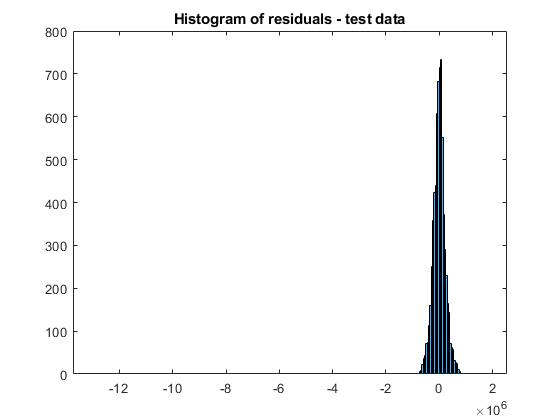The residual values have a few outliers.

`errs(isoutlier(errs,'grubbs'))`
```ans = 6×1 107 × 0.1788 -0.4688 -1.2981 0.1019 0.1122 0.1331 ```

### Analyze Using Tall Arrays

The `fitlm` function supports tall arrays for out-of-memory data, with some limitations. For tall data, `fitlm` returns a `CompactLinearModel` object that contains most of the same properties as a `LinearModel` object. The main difference is that the compact object is sensitive to memory requirements. The compact object does not have properties that include the data, or that include an array of the same size as the data. Therefore, some `LinearModel` object functions that require data do not work with a compact model. See Object Functions for the list of supported object functions. Also, see Tall Arrays for the usage notes and limitations of `fitlm` for tall arrays.

When you perform calculations on tall arrays, MATLAB® uses either a parallel pool (default if you have Parallel Computing Toolbox™) or the local MATLAB session. If you want to run the example using the local MATLAB session when you have Parallel Computing Toolbox, you can change the global execution environment by using the `mapreducer` function.

Assume that all the data in the datastore `ds` does not fit in memory. You can use `tall` instead of `readall` to read `ds`.

```NYCHousing2015 = tall(ds); ```

For this example, convert the in-memory table `NYCHousing2015` to a tall table by using the `tall` function.

`NYCHousing2015_t = tall(NYCHousing2015);`
```Starting parallel pool (parpool) using the 'local' profile ... Connected to the parallel pool (number of workers: 12). ```

Partition the data set into a training set and test set. When you use `cvpartition` with tall arrays, the function partitions the data set based on the variable supplied as the first input argument. For classification problems, you typically use the response variable (a grouping variable) and create a random stratified partition to get even distribution between training and test sets for all groups. For regression problems, this stratification is not adequate, and you can use the `'Stratify'` name-value pair argument to turn off the option.

In this example, specify the predictor variable `NYCHousing2015_t.borough` as the first input argument to make the distribution of boroughs roughly the same across the training and tests sets. For reproducibility, set the seed of the random number generator using `tallrng`. The results can vary depending on the number of workers and the execution environment for the tall arrays. For details, see Control Where Your Code Runs (MATLAB).

```tallrng('default') % For reproducibility c = cvpartition(NYCHousing2015_t.borough,"holdout",0.3); trainData_t = NYCHousing2015_t(training(c),:); testData_t = NYCHousing2015_t(test(c),:);```

Because `fitlm` returns a compact model object for tall arrays, you cannot improve the model using the `step` function. Instead, you can explore the model parameters by using the object functions and then adjust the model as needed. You can also gather a subset of the data into the workspace, use `stepwiselm` to iteratively develop the model in memory, and then scale up to use tall arrays. For details, see Model Development of Statistics and Machine Learning with Big Data Using Tall Arrays.

In this example, fit a linear regression model using the model formula of `newMdl2`.

`mdl_t = fitlm(trainData_t,newMdl2.Formula)`
```Evaluating tall expression using the Parallel Pool 'local': - Pass 1 of 1: 0% complete Evaluation 0% complete ```
```- Pass 1 of 1: Completed in 8 sec Evaluation completed in 12 sec ```
```mdl_t = Compact linear regression model: saleprice ~ [Linear formula with 26 terms in 8 predictors] Estimated Coefficients: Estimate SE tStat pValue ___________ __________ _________ __________ (Intercept) 1.0895e+06 6.1407e+05 1.7741 0.076059 borough_Brooklyn 4.075e+06 4.1225e+05 9.8848 5.6489e-23 borough_Manhattan 3.213e+07 1.3492e+07 2.3814 0.017258 borough_Queens 7.8533e+05 4.1507e+05 1.8921 0.058502 borough_Staten Island -3.4046e+05 4.6474e+05 -0.73258 0.46383 buildingclasscategory -2.0149e+06 2.8266e+05 -7.1281 1.0616e-12 residentialunits 1.1818e+06 1.8751e+05 6.3027 3.0019e-10 commercialunits 79902 40099 1.9926 0.046323 landsquarefeet -542.7 66.845 -8.1188 5.0523e-16 grosssquarefeet 174.04 13.81 12.603 3.0459e-36 yearbuilt -547.67 317.94 -1.7226 0.084984 MM 46489 33211 1.3998 0.16159 borough_Brooklyn:buildingclasscategory 5433.3 11992 0.45308 0.65049 borough_Manhattan:buildingclasscategory 1.8465e+05 1.0041e+05 1.8389 0.065946 borough_Queens:buildingclasscategory 19756 11674 1.6923 0.090611 borough_Staten Island:buildingclasscategory -1428.6 14972 -0.095417 0.92398 borough_Brooklyn:commercialunits -65209 40608 -1.6058 0.10833 borough_Manhattan:commercialunits 2.2004e+05 1.3161e+05 1.6719 0.094557 borough_Queens:commercialunits -64561 42053 -1.5352 0.12475 borough_Staten Island:commercialunits -1.5046e+05 58525 -2.5709 0.010152 borough_Brooklyn:landsquarefeet 0.028354 5.1115 0.0055471 0.99557 borough_Manhattan:landsquarefeet -108.39 79.386 -1.3653 0.17218 borough_Queens:landsquarefeet -9.1634 3.9482 -2.3209 0.020304 borough_Staten Island:landsquarefeet -20.871 3.6468 -5.7232 1.0646e-08 borough_Brooklyn:grosssquarefeet 40.01 10.469 3.8216 0.00013311 borough_Manhattan:grosssquarefeet -57.441 89.807 -0.63961 0.52244 borough_Queens:grosssquarefeet 70.971 10.664 6.6549 2.9277e-11 borough_Staten Island:grosssquarefeet 35.305 12.002 2.9416 0.0032704 borough_Brooklyn:yearbuilt -2021.5 213.29 -9.4779 2.9548e-21 borough_Manhattan:yearbuilt -16693 7045.3 -2.3694 0.017828 borough_Queens:yearbuilt -379.5 214.93 -1.7657 0.077468 borough_Staten Island:yearbuilt 190.07 238.97 0.79537 0.42641 buildingclasscategory:residentialunits 25916 5486.2 4.7238 2.3349e-06 buildingclasscategory:landsquarefeet -3.7408 2.0796 -1.7988 0.072065 buildingclasscategory:grosssquarefeet -45.55 5.0342 -9.048 1.6222e-19 buildingclasscategory:yearbuilt 1066.2 144.27 7.3902 1.5394e-13 residentialunits:landsquarefeet -0.5859 0.10591 -5.5319 3.2189e-08 residentialunits:grosssquarefeet 1.712 0.41925 4.0836 4.4565e-05 residentialunits:yearbuilt -640.18 94.263 -6.7914 1.1499e-11 commercialunits:grosssquarefeet -19.763 4.6031 -4.2933 1.7706e-05 landsquarefeet:grosssquarefeet -0.0080089 0.00086203 -9.2908 1.7275e-20 landsquarefeet:yearbuilt 0.30618 0.034782 8.8027 1.4713e-18 landsquarefeet:MM 0.53584 0.28203 1.8999 0.057462 yearbuilt:MM -22.47 17.123 -1.3122 0.18946 Number of observations: 15852, Error degrees of freedom: 15808 Root Mean Squared Error: 2.25e+05 R-squared: 0.275, Adjusted R-Squared: 0.274 F-statistic vs. constant model: 140, p-value = 0 ```

`mdl_t` is a `CompactLinearModel` object. `mdl_t` is not exactly the same as `newMdl2` because the partitioned training data set obtained from the tall table is not the same as the one from the in-memory data set.

You cannot use the `plotResiduals` function to create a histogram of the model residuals because `mdl_t` is a compact object. Instead, compute the residuals directly from the compact object and create the histogram using `histogram`.

```mdl_t_Residual = trainData_t.saleprice - predict(mdl_t,trainData_t); histogram(mdl_t_Residual)```
```Evaluating tall expression using the Parallel Pool 'local': - Pass 1 of 2: Completed in 3.6 sec - Pass 2 of 2: Completed in 1.6 sec Evaluation completed in 7.6 sec ```
`title("Histogram of residuals - train data")`Predict responses to the test data set `testData_t` by using `predict`.

`ypred_t = predict(mdl_t,testData_t);`

Plot the residual histogram of the test data set.

```errs_t = ypred_t - testData_t.saleprice; histogram(errs_t)```
```Evaluating tall expression using the Parallel Pool 'local': - Pass 1 of 2: Completed in 1.6 sec - Pass 2 of 2: Completed in 1.3 sec Evaluation completed in 5.4 sec ```
`title("Histogram of residuals - test data")`You can further assess the fitted model using the `CompactLinearModel` object functions. For an example, see Assess and Adjust Model of Statistics and Machine Learning with Big Data Using Tall Arrays.